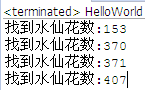控制流程系列教材 （七）- java 如何结束外部for循环 控制流程系列教材 （九）- 调试单步运行

## 练习-黄金分割点

姿势不对,事倍功半! 点击查看做练习的正确姿势## 答案-黄金分割点13分44秒 本视频采用html5方式播放，如无法正常播放，请将浏览器升级至最新版本，推荐火狐，chrome，360浏览器。 如果装有迅雷，播放视频呈现直接下载状态，请调整 迅雷系统设置-基本设置-启动-监视全部浏览器 (去掉这个选项)。 chrome 的 视频下载插件会影响播放，如 IDM 等，请关闭或者切换其他浏览器

public class HelloWorld { public static void main(String[] args) { // 寻找某两个数相除，其结果 离黄金分割点 0.618最近 // // 分母和分子不能同时为偶数 // 分母和分子 取值范围在[1-20] int range = 20; // 取值范围 float breakPoint = 0.618f; // 黄金分割点 float minDiff = 100; // 离黄金分割点的差值 int answerFenzi = 0; // 找到的分子 int answerFenmu = 0; // 找到的分母 for (int fenzi = 1; fenzi <= range; fenzi++) { for (int fenmu = 1; fenmu <= range; fenmu++) { // 分母和分子不能同时为偶数 if (0 == fenzi % 2 & 0 == fenmu % 2) continue; // 取值 float value = (float) fenzi / fenmu; // 取离黄金分割点的差值 float diff = value - breakPoint; // 绝对值 diff = diff < 0 ? 0 - diff : diff; // 找出最小的差值 if (diff < minDiff) { minDiff = diff; answerFenzi = fenzi; answerFenmu = fenmu; } } } System.out.println("离黄金分割点(" + breakPoint + ")最近的两个数相除是:" + answerFenzi + "/" + answerFenmu + "=" + ((float) answerFenzi / answerFenmu)); } }

## 练习-水仙花数

姿势不对,事倍功半! 点击查看做练习的正确姿势

1. 一定是3位数
2. 每一位的立方，加起来恰好是这个数本身，比如153=1*1*1+5*5*5+3*3*3

## 答案-水仙花数4分16秒 本视频采用html5方式播放，如无法正常播放，请将浏览器升级至最新版本，推荐火狐，chrome，360浏览器。 如果装有迅雷，播放视频呈现直接下载状态，请调整 迅雷系统设置-基本设置-启动-监视全部浏览器 (去掉这个选项)。 chrome 的 视频下载插件会影响播放，如 IDM 等，请关闭或者切换其他浏览器public class HelloWorld { public static void main(String[] args) { for (int i = 100; i < 1000; i++) { int baiwei = i / 100; int shiwei = i / 10 % 10; int gewei = i % 10; int cube = baiwei * baiwei * baiwei + shiwei * shiwei * shiwei + gewei * gewei * gewei; if (cube == i) { System.out.println("找到水仙花数:" + i); } } } }
```public class HelloWorld {
public static void main(String[] args) {

for (int i = 100; i < 1000; i++) {

int baiwei = i / 100;
int shiwei = i / 10 % 10;
int gewei = i % 10;
int cube = baiwei * baiwei * baiwei + shiwei * shiwei * shiwei + gewei * gewei * gewei;
if (cube == i) {
System.out.println("找到水仙花数:" + i);
}

}

}

}
```

## 练习-小学算术题

姿势不对,事倍功半! 点击查看做练习的正确姿势## 答案-小学算术题5分14秒 本视频采用html5方式播放，如无法正常播放，请将浏览器升级至最新版本，推荐火狐，chrome，360浏览器。 如果装有迅雷，播放视频呈现直接下载状态，请调整 迅雷系统设置-基本设置-启动-监视全部浏览器 (去掉这个选项)。 chrome 的 视频下载插件会影响播放，如 IDM 等，请关闭或者切换其他浏览器

public class HelloWorld { public static void main(String[] args) { int a = 0; int b = 0; int c = 0; int d = 0; for (a = -100; a <= 100; a++) { for (b = -100; b <= 100; b++) { for (c = -100; c <= 100; c++) { for (d = -100; d <= 100; d++) { if(a+b==8&&c-d==6&&a+c==14&&b+d==10){ System.out.println("a:"+a); System.out.println("b:"+b); System.out.println("c:"+c); System.out.println("d:"+d); } } } } } } }

 控制流程系列教材 （七）- java 如何结束外部for循环 控制流程系列教材 （九）- 调试单步运行

HOW2J公众号，关注后实时获知最新的教程和优惠活动，谢谢。2022-07-12 第三题 3 5 11 5
 zbmmm 关于 JAVA 基础-控制流程-综合练习 的提问 如题 ``` for (int i = 0 ;i < 100 ;i++){ for (int j = 0 ;j < 100 ;j++){ for (int m = 0 ;m < 100 ;m++){ for (int n = 0 ;n < 100 ;n++){ if (i + j == 8 && i + m == 14 && j + n ==10 && m - n ==6){ System.out.println("i=" + i + "j=" + j + "m=" + m + "n=" + n); } } } } } ```

1 个答案

lllfffzzz

public static void main(String[] args) { for (int i = 0; i<=8; i++) { int ii=8-i; for (int j = 6; j<=22; j++) { int jj=j-6; if (i+j==14 && ii+jj==10){ System.out.println(i+":"+ii+"\n"+j+":"+jj); } } } } }

2022-07-08
 YE_coding 关于 JAVA 基础-控制流程-综合练习 的提问 1、黄金分割 ```package test01; public class tset { public static void main(String[] args){ double target=0.618; int x,y,record_x = 0,record_y=0; double result=1; for (x=1;x<=20;x++){ for (y=1;y<=20;y++){ if (x%2!=0 || y%2!=0){ if (Math.abs((double) x/(double) y-target)

1 个答案

2022-07-08
2022-06-30 两个循环没有就执行了一遍
2022-04-14 大家看看